# Bayesian Rugby

I came across the following blog post on http://danielweitzenfeld.github.io/passtheroc/blog/2014/10/28/bayes-premier-league/ I quote from him, about his realization about Premier League Football - _It occurred to me that this problem is perfect for a Bayesian model. We want to infer the latent paremeters (every team's strength) that are generating the data we observe (the scorelines). Moreover, we know that the scorelines are a noisy measurement of team strength, so ideally, we want a model that makes it easy to quantify our uncertainty about the underlying strengths.

So I googled 'Bayesian football' and found this paper, called 'Bayesian hierarchical model for the prediction of football results.' The authors (Gianluca Baio and Marta A. Blangiardo) being Italian, though, the 'football' here is soccer.

In this post, I'm going to reproduce the first model described in the paper using pymc. While they used Seria A in their paper, I'm going to use the 2013-2014 Premier League.

Since I am a rugby fan I decide to apply the results of the paper Bayesian Football to the Six Nations.

## Acquiring the data

The first step was to acquire the data - which I created in a csv file from data I got on wikipedia and sports websites. To be honest a lot of this turned out to be manual entry. But this is fine for T=6 teams :)

We largely follow the code of the website cited above, with only a few small changes. We do less wrangling because I personally curated the data.

In :
%matplotlib inline
%config InlineBackend.figure_format = 'retina'

In :
import os
import math
import warnings
warnings.filterwarnings('ignore')

from IPython.display import Image, HTML
import numpy as np
import pandas as pd
import seaborn as sns
import matplotlib.pyplot as plt
import pymc # I know folks are switching to "as pm" but I'm just not there yet

In :
DATA_DIR = os.path.join(os.getcwd(), 'data/')


Now that we've set the right path, we import the data from a csv file and inspect it.

In :
data_file = DATA_DIR + 'results_2014.csv'

Out:
home_team away_team home_score away_score
0 Wales Italy 23 15
1 France England 26 24
2 Ireland Scotland 28 6
3 Ireland Wales 26 3
4 Scotland England 0 20

Like the tutorial I create an integer for the teams. I tried doing this a different way but this turned out to be the most efficient method

In :
teams = df.home_team.unique()
teams = pd.DataFrame(teams, columns=['team'])
teams['i'] = teams.index

Out:
team i
0 Wales 0
1 France 1
2 Ireland 2
3 Scotland 3
4 Italy 4
In :
teams

Out:
team i
0 Wales 0
1 France 1
2 Ireland 2
3 Scotland 3
4 Italy 4
5 England 5

Now that we have inspected the teams we can go through the 'merging' phase or 'joining'

In :
df = pd.merge(df, teams, left_on='home_team', right_on='team', how='left')
df = df.rename(columns = {'i': 'i_home'}).drop('team', 1)
df = pd.merge(df, teams, left_on='away_team', right_on='team', how='left')
df = df.rename(columns = {'i': 'i_away'}).drop('team', 1)

Out:
home_team away_team home_score away_score i_home i_away
0 Wales Italy 23 15 0 4
1 France England 26 24 1 5
2 Ireland Scotland 28 6 2 3
3 Ireland Wales 26 3 2 0
4 Scotland England 0 20 3 5
In :
observed_home_goals = df.home_score.values
observed_away_goals = df.away_score.values
home_team = df.i_home.values
away_team = df.i_away.values
num_teams = len(df.i_home.drop_duplicates())
num_games = len(home_team)


Now, that we've extracted the data into arrays, so that pymc can work with it. Note that each of the arrays (observed_home_goals, observed_away_goals, home_team, away_team) are the same length, and that the ith entry of each refers to the same game - we can do some sanity checks. A simple one is 'what is the num of teams'?

In :
num_teams

Out:
6

In :
df

Out:
home_team away_team home_score away_score i_home i_away
0 Wales Italy 23 15 0 4
1 France England 26 24 1 5
2 Ireland Scotland 28 6 2 3
3 Ireland Wales 26 3 2 0
4 Scotland England 0 20 3 5
5 France Italy 30 10 1 4
6 Wales France 27 6 0 1
7 Italy Scotland 20 21 4 3
8 England Ireland 13 10 5 2
9 Ireland Italy 46 7 2 4
10 Scotland France 17 19 3 1
11 England Wales 29 18 5 0
12 Italy England 11 52 4 5
13 Wales Scotland 51 3 0 3
14 France Ireland 20 22 1 2

Now we do some transformation of the scores - I find when it comes to fitting such data to Bayesian models this is a decent step.

In :
g = df.groupby('i_away')
att_starting_points = np.log(g.away_score.mean())
g = df.groupby('i_home')
def_starting_points = -np.log(g.away_score.mean())


# The model.

The league is made up by a total of T= 6 teams, playing each other once in a season. We indicate the number of points scored by the home and the away team in the g-th game of the season (15 games) as $y_{g1}$ and $y_{g2}$ respectively.

The vector of observed counts $\mathbb{y} = (y_{g1}, y_{g2})$ is modelled as independent Poisson: $y_{gi}| \theta_{gj} \tilde\;\; Poisson(\theta_{gj})$ where the theta parameters represent the scoring intensity in the g-th game for the team playing at home (j=1) and away (j=2), respectively.

We model these parameters according to a formulation that has been used widely in the statistical literature, assuming a log-linear random effect model: $$log \theta_{g1} = home + att_{h(g)} + def_{a(g)}$$ $$log \theta_{g2} = att_{a(g)} + def_{h(g)}$$ the parameter home represents the advantage for the team hosting the game and we assume that this effect is constant for all the teams and throughout the season. In addition, the scoring intensity is determined jointly by the attack and defense ability of the two teams involved, represented by the parameters att and def, respectively. In line with the Bayesian approach, we have to specify some suitable prior distributions for all the random parameters in our model. The variable $home$ is modelled as a fixed effect, assuming a standard flat prior distribution. We use the notation of describing the Normal distribution in terms of mean and the precision. $home \tilde\; Normal(0,0.0001)$

Conversely, for each t = 1, ..., T, the team-specific effects are modelled as exchangeable from a common distribution: $att_t \tilde\; Normal(\mu_{att}, \tau_{att})$ and $def_t \tilde\; Normal(\mu_{def}, \tau_{def})$

Note that they're breaking out team strength into attacking and defending strength. A negative defense parameter will sap the mojo from the opposing team's attacking parameter.

I made two tweaks to the model. It didn't make sense to me to have a $\mu_{att}$ when we're enforcing the sum-to-zero constraint by subtracting the mean anyway. So I eliminated $\mu_{att}$ and $\mu_{def}$

Also because of the sum-to-zero constraint, it seemed to me that we needed an intercept term in the log-linear model, capturing the average goals scored per game by the away team. This we model with the following hyperprior. $$intercept \tilde\; Normal(0, 0.001)$$

In :
#hyperpriors
home = pymc.Normal('home', 0, .0001, value=0)
tau_att = pymc.Gamma('tau_att', .1, .1, value=10)
tau_def = pymc.Gamma('tau_def', .1, .1, value=10)
intercept = pymc.Normal('intercept', 0, .0001, value=0)

In :
#team-specific parameters
atts_star = pymc.Normal("atts_star",
mu=0,
tau=tau_att,
size=num_teams,
value=att_starting_points.values)
defs_star = pymc.Normal("defs_star",
mu=0,
tau=tau_def,
size=num_teams,
value=def_starting_points.values)

In :
# trick to code the sum to zero contraint
@pymc.deterministic
def atts(atts_star=atts_star):
atts = atts_star.copy()
atts = atts - np.mean(atts_star)
return atts

@pymc.deterministic
def defs(defs_star=defs_star):
defs = defs_star.copy()
defs = defs - np.mean(defs_star)
return defs

@pymc.deterministic
def home_theta(home_team=home_team,
away_team=away_team,
home=home,
atts=atts,
defs=defs,
intercept=intercept):
return np.exp(intercept +
home +
atts[home_team] +
defs[away_team])

@pymc.deterministic
def away_theta(home_team=home_team,
away_team=away_team,
home=home,
atts=atts,
defs=defs,
intercept=intercept):
return np.exp(intercept +
atts[away_team] +
defs[home_team])

home_points = pymc.Poisson('home_points',
mu=home_theta,
value=observed_home_goals,
observed=True)
away_points = pymc.Poisson('away_points',
mu=away_theta,
value=observed_away_goals,
observed=True)

mcmc = pymc.MCMC([home, intercept, tau_att, tau_def,
home_theta, away_theta,
atts_star, defs_star, atts, defs,
home_points, away_points])
map_ = pymc.MAP( mcmc )
map_.fit()
mcmc.sample(200000, 40000, 20)

 [-----------------100%-----------------] 200000 of 200000 complete in 252.3 sec


# Diagnostics

Let's see if/how the model converged. The home parameter looks good, and indicates that home field advantage amounts to goals per game at the intercept.

We can see that it converges just like the model for the Premier League in the other tutorial.

I wonder and this is left as a question if all field sports have models of this form that converge.

In :
pymc.Matplot.plot(home)

Plotting home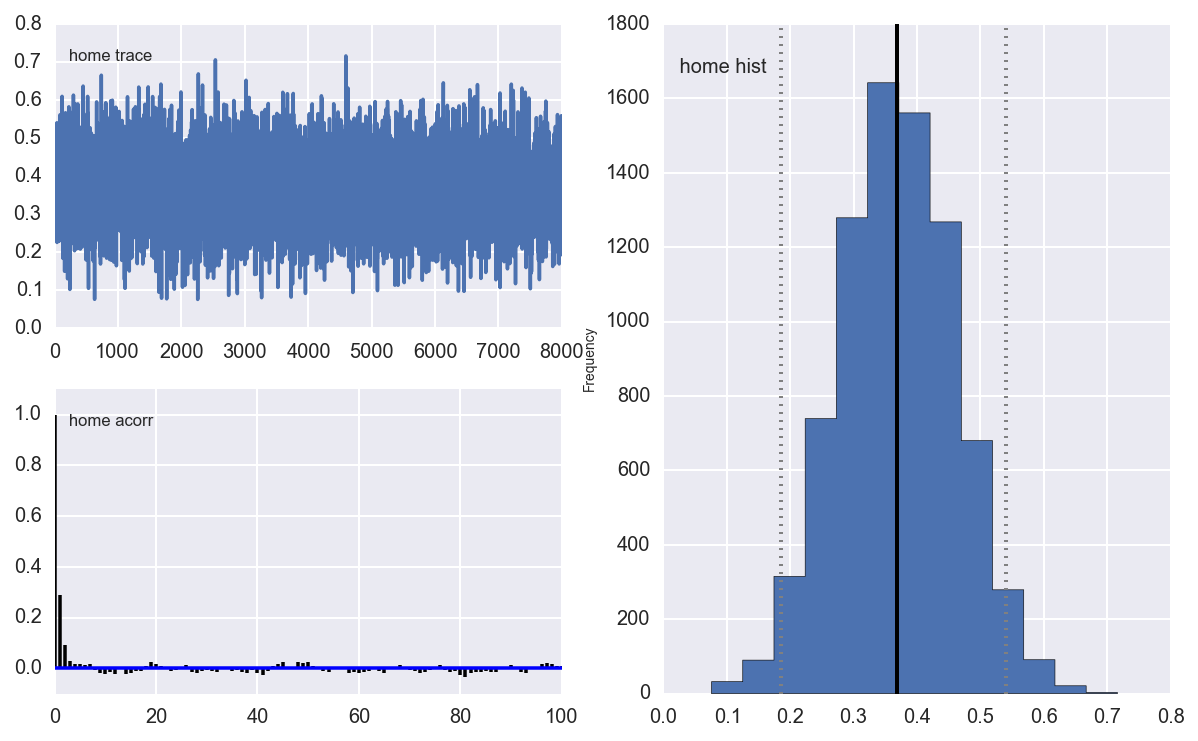In :
pymc.Matplot.plot(intercept)

Plotting intercept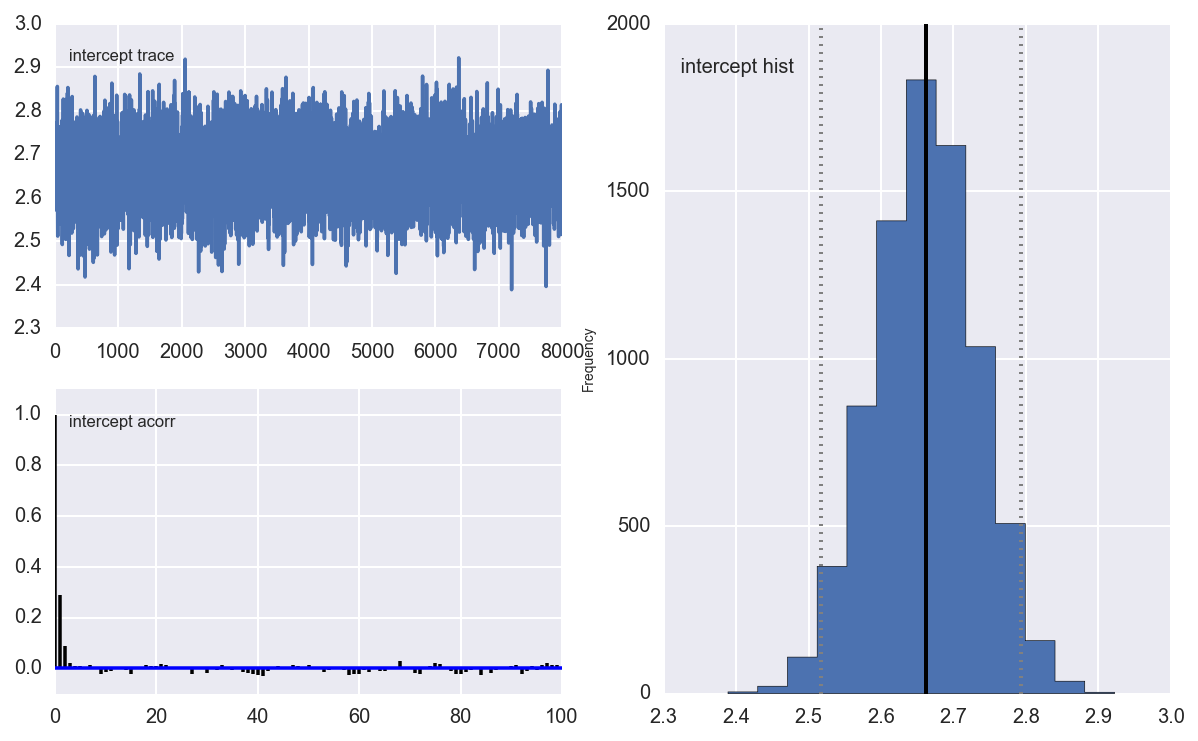In :
pymc.Matplot.plot(tau_att)

Plotting tau_att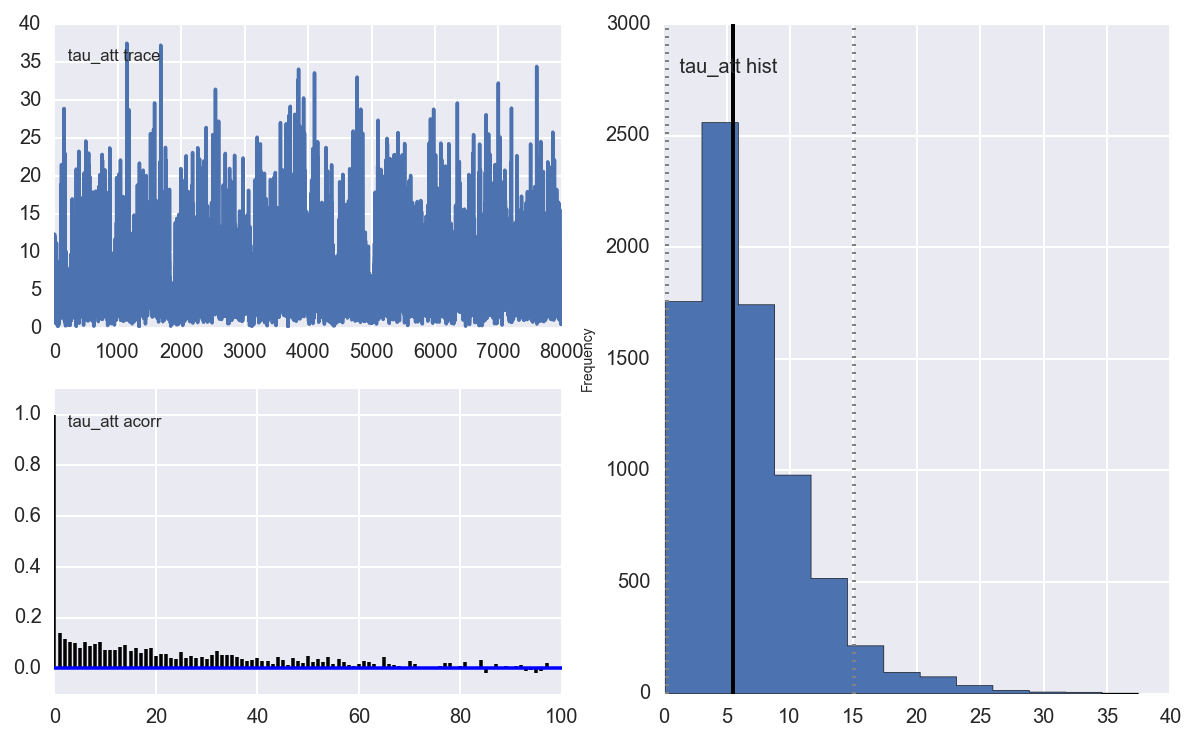In :
pymc.Matplot.plot(tau_def)

Plotting tau_def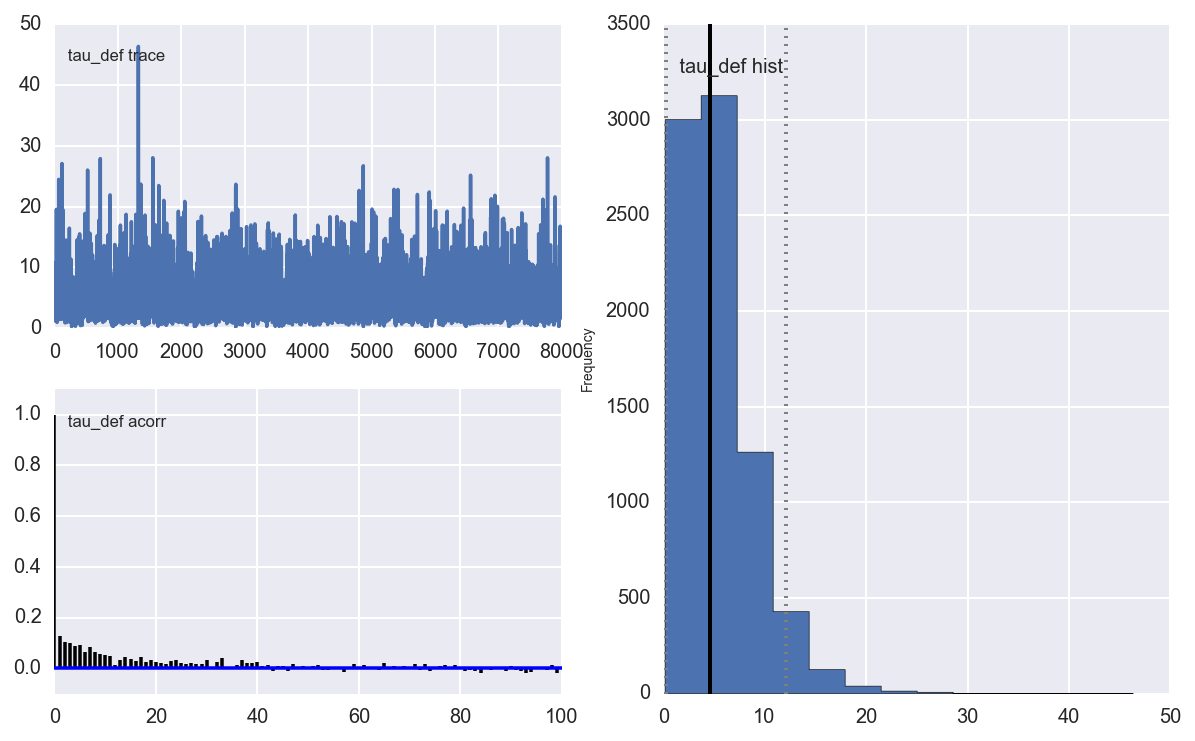It looks like we have decent parameters and that the intercept was a good idea. I'm not so sure about how autocorrelated the Tau terms are but that is for me me to brush up on my Bayesian models.

# Simulation

Now we pull in some observed data (i.e. the table from last year) and include some remarks about Qualification

In :
observed_season = DATA_DIR + 'table_2014.csv'
df_observed.loc[df_observed.QR.isnull(), 'QR'] = ''

In :
def simulate_season():
"""
Simulate a season once, using one random draw from the mcmc chain.
"""
num_samples = atts.trace().shape
draw = np.random.randint(0, num_samples)
atts_draw = pd.DataFrame({'att': atts.trace()[draw, :],})
defs_draw = pd.DataFrame({'def': defs.trace()[draw, :],})
home_draw = home.trace()[draw]
intercept_draw = intercept.trace()[draw]
season = df.copy()
season = pd.merge(season, atts_draw, left_on='i_home', right_index=True)
season = pd.merge(season, defs_draw, left_on='i_home', right_index=True)
season = season.rename(columns = {'att': 'att_home', 'def': 'def_home'})
season = pd.merge(season, atts_draw, left_on='i_away', right_index=True)
season = pd.merge(season, defs_draw, left_on='i_away', right_index=True)
season = season.rename(columns = {'att': 'att_away', 'def': 'def_away'})
season['home'] = home_draw
season['intercept'] = intercept_draw
season['home_theta'] = season.apply(lambda x: math.exp(x['intercept'] +
x['home'] +
x['att_home'] +
x['def_away']), axis=1)
season['away_theta'] = season.apply(lambda x: math.exp(x['intercept'] +
x['att_away'] +
x['def_home']), axis=1)
season['home_goals'] = season.apply(lambda x: np.random.poisson(x['home_theta']), axis=1)
season['away_goals'] = season.apply(lambda x: np.random.poisson(x['away_theta']), axis=1)
season['home_outcome'] = season.apply(lambda x: 'win' if x['home_goals'] > x['away_goals'] else
'loss' if x['home_goals'] < x['away_goals'] else 'draw', axis=1)
season['away_outcome'] = season.apply(lambda x: 'win' if x['home_goals'] < x['away_goals'] else
'loss' if x['home_goals'] > x['away_goals'] else 'draw', axis=1)
season = season.join(pd.get_dummies(season.home_outcome, prefix='home'))
season = season.join(pd.get_dummies(season.away_outcome, prefix='away'))
return season

def create_season_table(season):
"""
Using a season dataframe output by simulate_season(), create a summary dataframe with wins, losses, goals for, etc.

"""
g = season.groupby('i_home')
home = pd.DataFrame({'home_goals': g.home_goals.sum(),
'home_goals_against': g.away_goals.sum(),
'home_wins': g.home_win.sum(),
'home_losses': g.home_loss.sum()
})
g = season.groupby('i_away')
away = pd.DataFrame({'away_goals': g.away_goals.sum(),
'away_goals_against': g.home_goals.sum(),
'away_wins': g.away_win.sum(),
'away_losses': g.away_loss.sum()
})
df = home.join(away)
df['wins'] = df.home_wins + df.away_wins
df['losses'] = df.home_losses + df.away_losses
df['points'] = df.wins * 2
df['gf'] = df.home_goals + df.away_goals
df['ga'] = df.home_goals_against + df.away_goals_against
df['gd'] = df.gf - df.ga
df = pd.merge(teams, df, left_on='i', right_index=True)
df = df.sort_index(by='points', ascending=False)
df = df.reset_index()
df['position'] = df.index + 1
df['champion'] = (df.position == 1).astype(int)
df['relegated'] = (df.position > 5).astype(int)
return df

def simulate_seasons(n=100):
dfs = []
for i in range(n):
s = simulate_season()
t = create_season_table(s)
t['iteration'] = i
dfs.append(t)
return pd.concat(dfs, ignore_index=True)


We simulate 10000 seasons. And then for fun (and because I am Irish we look at Ireland)

In :
simuls = simulate_seasons(10000)

In :
ax = simuls.points[simuls.team == 'Ireland'].hist(figsize=(7,5))
median = simuls.points[simuls.team == 'Ireland'].median()
ax.set_title('Ireland: 2013-14 points, 1000 simulations')
ax.plot([median, median], ax.get_ylim())
plt.annotate('Median: %s' % median, xy=(median + 1, ax.get_ylim()-10))

Out:
<matplotlib.text.Annotation at 0xa952870c>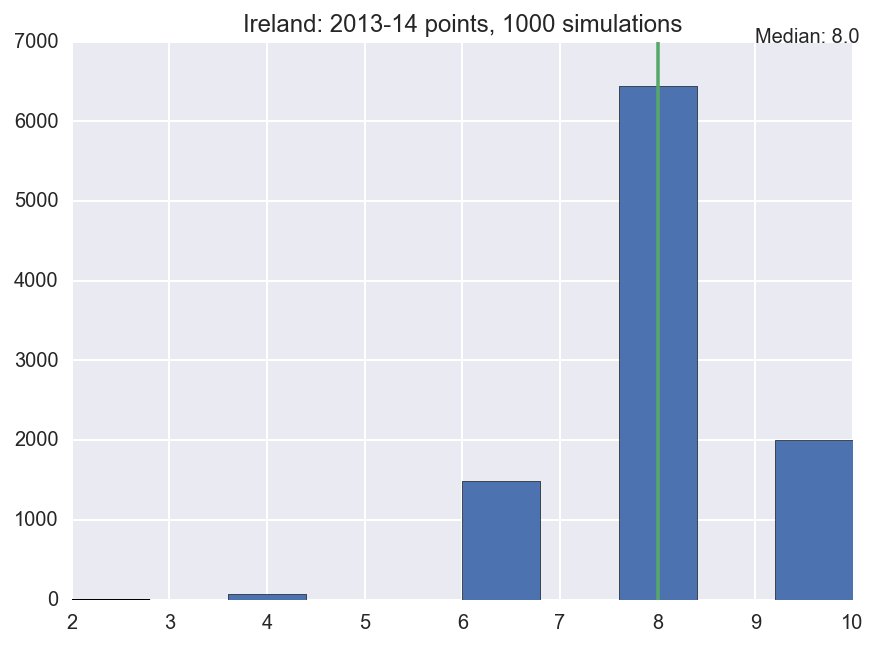In :
ax = simuls.gf[simuls.team == 'Ireland'].hist(figsize=(7,5))
median = simuls.gf[simuls.team == 'Ireland'].median()
ax.set_title('Ireland: 2013-14 points for, 1000 simulations')
ax.plot([median, median], ax.get_ylim())
plt.annotate('Median: %s' % median, xy=(median + 1, ax.get_ylim()-10))

Out:
<matplotlib.text.Annotation at 0xa949f5cc>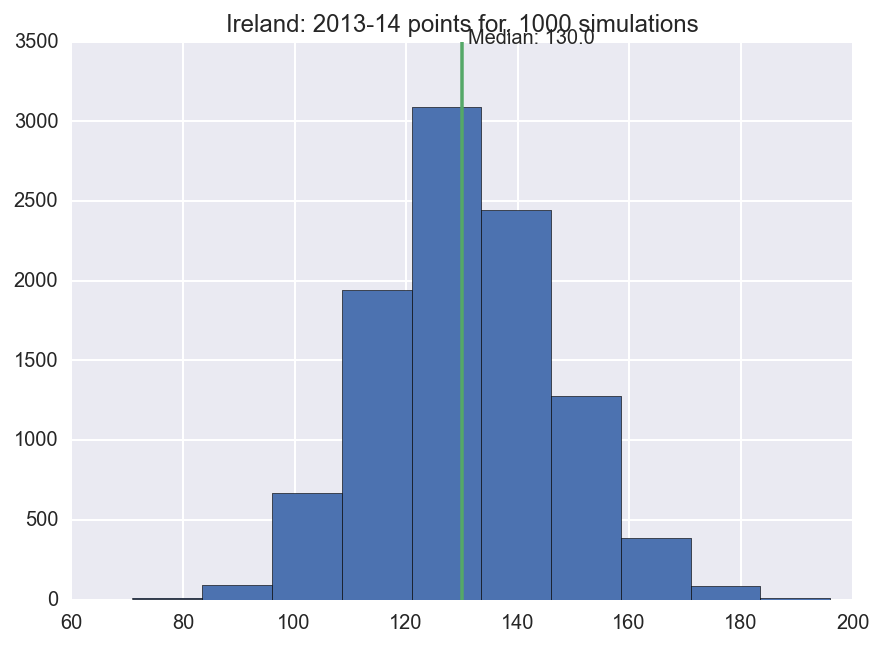So those numbers seem reasonable from my historical knowledge of the Six Nations. Now as a final act I'll do an a simulation of who will win in alternative Universes

In :
g = simuls.groupby('team')
df_champs = pd.DataFrame({'percent_champs': g.champion.mean()})
df_champs = df_champs.sort_index(by='percent_champs')
df_champs = df_champs[df_champs.percent_champs > .05]
df_champs = df_champs.reset_index()

fig, ax = plt.subplots(figsize=(8,6))
ax.barh(df_champs.index.values, df_champs.percent_champs.values)

for i, row in df_champs.iterrows():
label = "{0:.1f}%".format(100 * row['percent_champs'])
ax.annotate(label, xy=(row['percent_champs'], i), xytext = (3, 10), textcoords = 'offset points')
ax.set_ylabel('Team')
ax.set_title('% of Simulated Seasons In Which Team Finished Winners')
_= ax.set_yticks(df_champs.index + .5)
_= ax.set_yticklabels(df_champs['team'].values)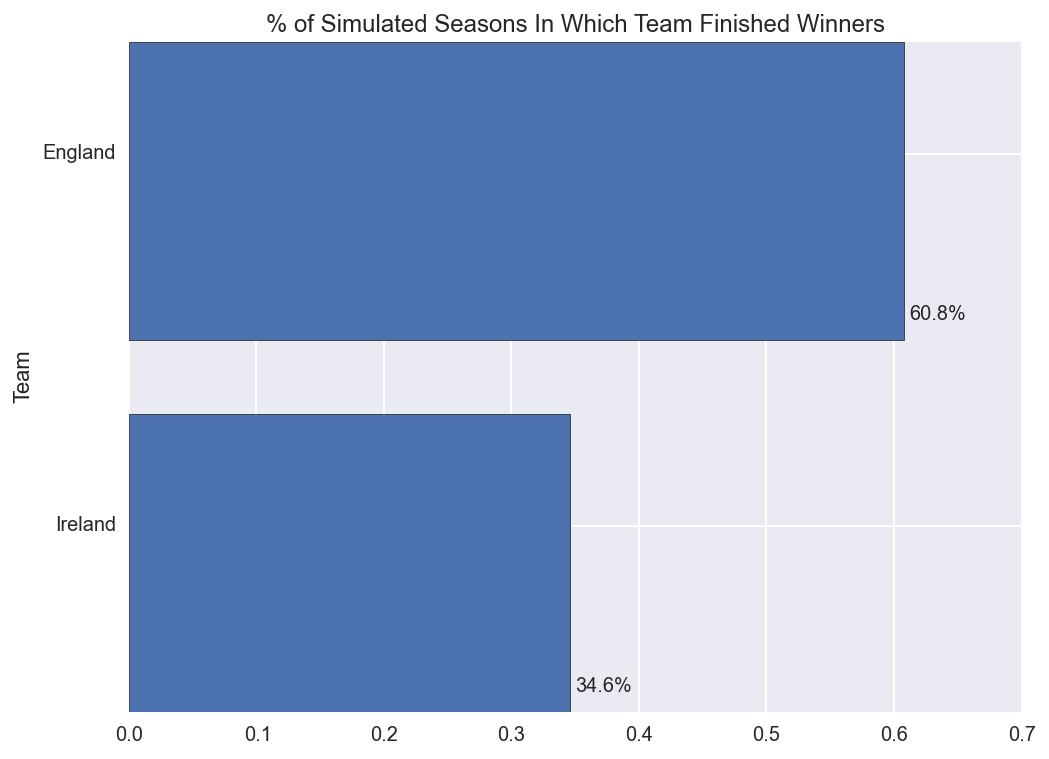Unfortunately it seems that in most of the Universes England come top of the Six Nations. And as an Irish man this is firm proof that I put Mathematical rigour before patriotism :)

This is a reasonable result, and I hope it proved a nice example of Bayesian models in Rugby Analytics.